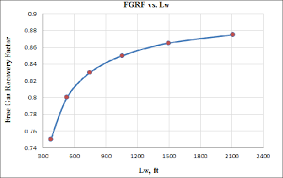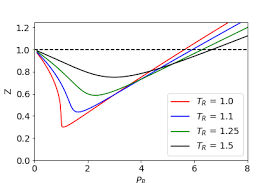## How to Calculate and Solve for Gas Recovery Factor of a Reservoir Fluid Flow | The Calculator EncyclopediaThe image above represents a gas recovery factor.

To compute for the gas recovery factor, three essential parameters are needed and these parameters are initial pressure(Pi), initial compressibility factor (Ziand P/Z Ratio (P/Z).

The formula for calculating the gas recovery factor:

RFG = 1 – P/Z.Pi / Zi

Where;

RFG = Gas Recovery Factor (P, Z)
Pi = Initial Pressure
Zi = Initial Compressibility Factor
P/Z = P/Z Ratio

Let’s solve an example;
Find the gas recovery factor when the initial pressure is 12, the initial compressibility factor is 7 and the P/Z ratio is 36.

This implies that;

Pi = Initial Pressure = 12
Zi = Initial Compressibility Factor = 7
P/Z = P/Z Ratio = 36

RFG = 1 – P / Z.Pi / Zi
RFG = 1 – 36[12 / 7]
RFG = 1 – 36[1.714]
RFG = 1 – 61.714
RFG = -60.714

Therefore, the gas recovery factor is -60.714.

## How to Calculate and Solve for Pressure and Initial Compressibility Factor Ratio of a Reservoir Fluid Flow | The Calculator EncyclopediaThe image above represents pressure and initial compressibility factor ratio (P/Z Ratio).

To compute for the pressure and initial compressibility factor ratio, four essential parameters are needed and these parameters are initial pressure (Pi), Initial Compressibility Factor (Zi), Cumulative Gas Production (GP) and initial gas in place (G).

The formula for calculating the P/Z Ratio:

P/Z = Pi / Zi (1 – Gp / G)

Where;

P/Z = P/Z Ratio
Pi = Initial Pressure
Zi = Initial Compressibility Factor
GP = Cumulative Gas Production
G = Initial Gas in Place

Let’s solve an example;
Given that the initial pressure is 20, the initial compressibility factor is 28, the cumulative gas production is 30 and the initial gas in place is 34.
Find the pressure and initial compressibility factor ratio (P/Z Ratio)?

This implies that;

Pi = Initial Pressure = 20
Zi = Initial Compressibility Factor = 28
GP = Cumulative Gas Production  = 30
G = Initial Gas in Place = 34

P/Z = Pi / Zi (1 – Gp / G)
P/Z = 20 / 28 (1 – 30 / 34)
P/Z = 20 / 28 (1 – 0.88)
P/Z = 20 / 28 (0.117)
P/Z = 0.714(0.117)
P/Z = 0.084

Therefore, the P/Z Ratio is 0.084.

Calculating for Initial Pressure when P/Z Ratio, Initial Compressibility Factor, Cumulative Gas Pressure and Initial Gas In Place is Given.

Pi = P/Z x Zi / (1 – Gp / G)

Where;

Pi = Initial Pressure
P/Z = P/Z Ratio
Zi = Initial Compressibility Factor
GP = Cumulative Gas Production
G = Initial Gas in Place

Let’s solve an example;
Given that the P/Z Ratio is 50, the initial compressibility factor is 18, the cumulative gas production is 25 and the initial gas in place is 32.
Find the initial pressure ?

This implies that;

P/Z = P/Z Ratio = 50
Zi = Initial Compressibility Factor = 18
GP = Cumulative Gas Production = 25
G = Initial Gas in Place = 32

Pi = P/Z x Zi / (1 – Gp / G)
Pi = 50 x 18 / (1 – 25 / 32)
Pi = 900 / (1 – 0.78125)
Pi = 900 / (0.21875)
Pi = 4114.28

Therefore, the Initial Pressure is 4114.28.

Calculating for Initial Compressibility Factor when P/Z Ratio, Initial Pressure, Cumulative Gas Pressure and Initial Gas In Place is Given.

Zi = Pi (1 – Gp / G) / P/Z

Where;

Zi = Initial Compressibility Factor
Pi = Initial Pressure
P/Z = P/Z Ratio
GP = Cumulative Gas Production
G = Initial Gas in Place

Let’s solve an example;
Given that the P/Z Ratio is 40, the initial pressure is 18, the cumulative gas production is 15 and the initial gas in place is 22.
Find the initial compressibility factor ?

This implies that;

Pi = Initial Pressure = 18
P/Z = P/Z Ratio = 40
GP = Cumulative Gas Production = 15
G = Initial Gas in Place = 22

Zi = Pi (1 – Gp / G) / P/Z
Zi = 18 (1 – 15 / 22) / 40
Zi = 18 (1 – 0.681) / 40
Zi = 18 (0.319) / 40
Zi = 5.742 / 40
Zi = 0.1435

Therefore, the initial compressibility factor is  0.1435.

## Mathematical Ratios Can Be Solved By Nickzom Calculator – The Calculator Encyclopedia

According to Math Is Fun,

A ratio says how much of one thing there is compared to another thing.

According to Wikipedia,

A ratio may be considered as an ordered pair of numbers, as a fraction with the first number in the numerator and the second as denominator, or as the value denoted by this fraction. Ratios of counts, given by (non-zero) natural numbers, denote positive rational numbers, and as such may occasionally result in natural numbers.

According to WikiHow,

Ratios are mathematical expressions that compare two or more numbers. They can compare absolute quantities and amounts or can be used to compare portions of a larger whole. Ratios can be calculated and written in several different ways, but the principles guiding the use of ratios are universal to all.

Nickzom Calculator+ (Professional Version) – The Calculator Encyclopedia solves ratio in mathematics for any number of fractional parts.For the calculator encyclopedia to be able to solve and present you a ratio. you must have to know the number of fractional parts you desire to compute the ratio for.